Functions - Domain and Range; Composition

Ex 1 Find the domain and range for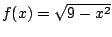.

Solis defined for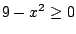or, and solving this inequality gives, so the domain is.

Sincegivesor, the graph ofis the top half of the circle; so the range of f is the set of all y-coordinates of points on this semicircle, which is the interval.

Ex 2 If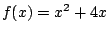and, findand.

Sol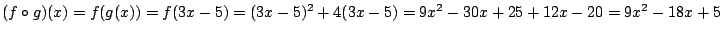, and.

Pr 1 Find the domain and range for.

Pr 2 Find the domain and range for.

Pr 3 Find the domain and range for.

Pr 4 Find the domain for.

Pr 5 If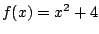and, findand.

Pr 6 If, find functions f and g (different from h) such that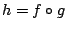.

Pr 7 If, find a function f such that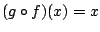.

Pr 8 Find the domain for.

Pr 9 Find the domain for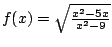.

Go to Solutions.